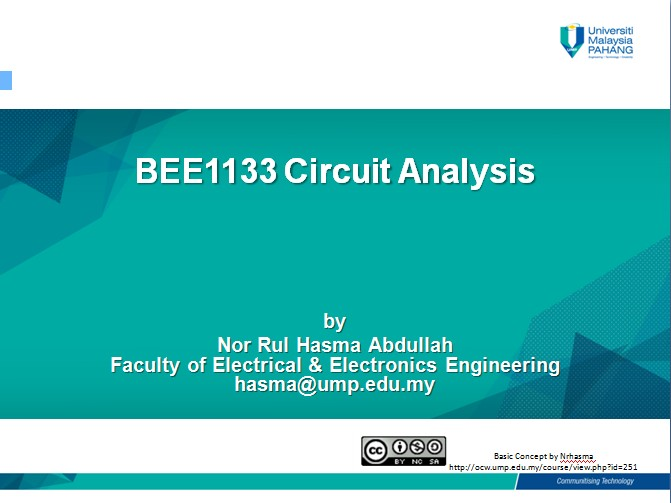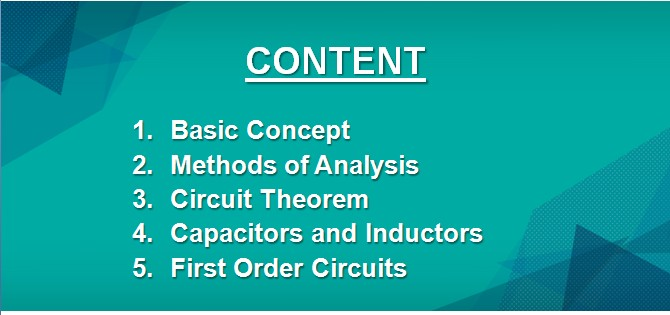## Topic outline

###Synopsis

This course introduces the basic concepts and engineering methods of DC circuit analysis. The contents include Ohm's Law, Kirchhoff's Law, series and parallel circuits, Mesh and Nodal analysis, Superposition, Source Transformation, Thevenin’s and Norton’s theorems, Capacitor, Inductor and responses of First Order circuits.

### Course Outcomes

At the end of this course student should be able to:

(i) Attribute the basic concepts of electrical quantities by using basic circuit laws (Ohm's law and Kirchhoff's law) and simplification of resistive circuits
(ii) Analyze DC circuit problems using circuit theorem, nodal analysis and mesh analysis
(iii) Attribute the basic concepts of capacitance and inductance and analyze the characteristic of natural and step response in first order circ uits
(iv) Construct DC electric circuits to apply the concept of electrical quantities and verify circuit theorems
(v) Demonstrate the role of individual in the team to achieve task completion

### References

1.   C. Alexander and M. Sadiku, “Fundamentals of Electric Circuits”, 4th ed., McGraw-Hill, 2008.

2.   J. Nilsson and S. Riedel, “Electric Circuits”, 8th ed., Prentice Hall, 2008.

3.   R. Dorf and J. Svoboda, "Introduction to Electric Circuits", 8th ed., John Wiley & Sons, 2010.

•Forum
• ### Topic 1

#### Chapter 1A: Basic Concept

1.1        Introduction of circuit analysis1.2        Electrical quantities: Systems of units, charge, current, voltage, power and energy
1.3        Circuit elements: Passive and active elements, independent and dependent sources

•• ### Topic 2

#### Chapter 1B: Basic Concept

2.1        Ohm’s Law and Kirchhoff's Law2.2        Nodes, branches and loops2.3        Resistive circuit: Series, parallel circuits and combination circuits

••File
• ### Topic 3

#### Chapter 1C: Basic Concept

3.1        Principles of voltage division and current division3.2        Delta-wye transformation

•• ### Topic 4

#### Chapter 2A: Methods of Analysis (DC Circuits)

4.1        Nodal Analysis4.2       Nodal analysis with voltage sources: Supernode


•• ### Topic 5

#### Chapter 2B: Methods of Analysis (DC Circuits)

5.1        Mesh Analysis5.2        Mesh Analysis with current sources: Supermesh5.3        Nodal versus Mesh Analysis

••File
• ### Topic 6

#### Chapter 3A: Circuit Theorem (DC Circuits)

6.1        Superposition Principles6.2       Source Transformation


•• ### Topic 7

#### Chapter 3B: Circuit Theorem (DC Circuits)

7.1        Thevenin's and Norton's Theorem (Independent and Dependent source)7.2        Maximum Power Transfer

••File
• ### Topic 8

#### Chapter 4: Capacitors and Inductors

8.1        Series and Parallel Capacitors8.2       Series and Parallel Inductors


••File
• ### Topic 9

#### Chapter 5A: First Order Circuits

9.1        Introduction to energy storage elements9.2        The source-free RC circuit
9.3        The source-free RL circuit9.4        Unit step function

••File
• ### Topic 10

#### Chapter 5B: First Order Circuits

10.1        Step response of an RC Circuit10.2        Step response of an RL Circuit

•### Labsheet

1. Laboratory 1: Ohm's Law and Kirrchoff's Law (Laboratory report)2. Laboratory 2: Nodal and Mesh Analysis (Technical Skill)
••• ### Past Year Final Examination Questions

•••